Výsledky výzkumu a další informace nejenz oblasti přístupových telekomunikačních sítí.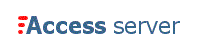ISSN 1214-9675
Server vznikl za podpory Grantové agentury ČR.
20. ročník
 Dnešní datum: 13. 08. 2022 Hlavní stránka | Seznam rubrik | Ke stažení | Odkazy

Doporučujeme
Knihu o FTTx

Matlab server - on-line výpočty a simulace

E-learning - on-line kurzy

Kontakt
KTT FEL ČVUT
Napište nám

Redakční rada - pokyny pro autory a recenzenty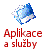#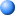Network Tomography Overview and Botnet Network Estimation, Part I.

Vydáno dne 23. 06. 2015 (7618 přečtení)

This article discusses about network tomography technology and intention to use such methods in combination with other techniques to estimate the appearance of a botnet network.

Abstract: The control of network availability uses a lot of technical methods to provide an overview about the security and quality of services. This article discusses about network tomography technology and intention to use such methods in combination with other techniques to estimate the appearance of a botnet network. This paper’s concept presents a shortened first part of research writing and proposes and brings an idea. The second part with practical tests will follow.

Keywords: botnet; genetics; measurement; network tomography; optimization.

### INTRODUCTION

Network behavior and adaptability to a demanded data transfer is under the focus of institutions which provide connectivity, security and also services to maintain almost 99,99% network usability. As the Internet of Everything (IoE), Internet of Things (IoT), Software Defined Network (SDN), other sophisticated platforms, protocols and, of course new cyber security laws are coming, the field of Network Tomography (NT) is growing. In addition, the computing power of equipment continues to increase, along with the development of new storage devices. The first significant mention of Network Tomography was in 1996. This term was used by Vardi . His intention was to capture the relationship between origin destination matrix (OD) estimation through link counts II-A.This article has also been inspired by examples and on-line tools; such as The Center for Applied Internet Data Analysis (CAIDA) , which collects a lot of tools provided by researchers or RIPE Labs ; to analyze which techniques and algorithms are mostly used to recognize a network flow, bottlenecks etc. Generally, all of them use passive or active access to measure, parse and evaluate data flow. Modern techniques add methods such as Symbolic Regression, Bayesian Networks, SVN and Gene Algorithms. OD estimation uses techniques as are Gaussian Model, Maximum Likelihood Estimation (MLE), Iterative Proportional Fitting (IPF), Maximum Pseudo-Likelihood Estimation (MPLE) or Partial Measurement (PM).

### NETWORK TOMOGRAPHY OVERVIEW

Network Tomography is a discipline that studies the internal behavior and characteristics of a network by external sources. These sources include endpoints, edge nodes and network probes, computers, mobiles, routers and other specialized equipment. All of these can provide data to use for analysis. For an example, an illustration of a network is shown in Fig. 1. The blue nodes cooperate and can provide some data to analyze it. The red nodes cannot be set up to estimate some data for various reason. NT proclaims that it is possible to effectively map the data path, capacity, quality, attacks, outward and so on by using this data information, if they are passively stored or actively real-time examined. For example, when generating certain selected probe traffic. We can say that these endpoints are kind of traffic monitors. What the proper monitors are able to do and what they are able to implement is another question. The basic classes of NT can be considered as loss and delay tomography. The extended classes are behavior, typification, matrices representation.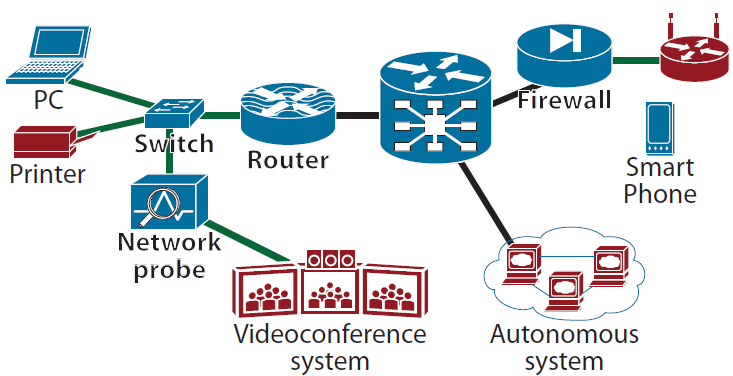Fig. 1: Network Ilustration.

A. Graph Theorem in Network Tomography

The bases of the NT theorem is given by the widely knowing graph theorem. Let graph Gi = (ν, ε), where the nodes ν represent equipment, with vertex set ν = {1, 2, 3,...|ν|} and ε represent the links among those equipment, edge from set ε ⊆ ν × ν. Then, (ν,ν') ∈ ε denotes a directed edge PG and δG(ν,ν') the shortest edge PδG from ν to ν'. Let be assumed connected graph, not incoherent. The graph, as is shown in Fig. 2, contain one edge for each node to node communication and one vertex for each node.Fig. 2: NT graph representation.

For example, let multicast delay estimation, when a tested frame is sent from node ν4 to node ν1 and ν3, where the delay is observed at the receivers only,the problem to infer the distribution of the internal links delay ε1, ε2, ε3 be as is shown in Equation (1),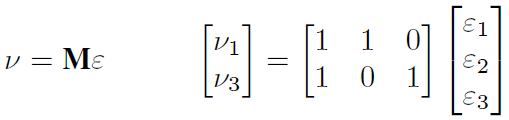(1)

where numbers 1 in matrix M express the path of probes.

### MULTI-OBJECTIVE OPTIMIZATION QUICK OVERVIEW

Multi-Objective Optimization (MOO) given the multiple fitness function (objectives) or goals, is able to find optimal solutions with respect to all criteria simultaneously, as discussed by Riccardo Poli et al. , for example. This article describes many examples of MOO usage. Generally, optimization operated such that if a problem is optimized, finds a set of decision variables. Those variables satisfied constraints and a vector function is optimized simultaneously. Such vector, which include elements, express the objective function of all decision and it leads to a non unique solution.

### DISCUSSION OF INTENT AND PROBLEM

Our aim is to use the knowledge of network tomography, in combination with multi-objective optimization in order to detect the appearance of a botnet network. We are doing this in such a context that after knowing this type of network it would be possible to isolate the reproductive behavior of a botnet code into small areas, without the possibility of further spread1. It means that the network probes will be used in real time as the monitors of patterns, and based on the NT identification and prediction of a botnet network would to isolation operation such blockage on predicted network elements.

1The two basic types of botnet network exists. Centralized and peer-to-peer (P2P) botnet. As is the first type more controllable, the second is not. The first is created by a root and spreads or connect contested node by a tree connection. The second type creates a mash topology, it means, it doesn’t use a centralized root-server. Every node in P2P can act as a root. From such point of view it is a lot harder to stop this kind of botnet.

A. Proposed intention of use the graph theory

Be graph theory adopted by NT and used in its simple form. For our intention let lists Y = {y(1), ..., y(N)}, where N = {G1, G2, ..., Gi | Gi ≈ Gi+1} and y(n) = {y1(n), ..., y|ν|(n)} associated with the coefficients ai = {0, 1}|ν| . Let for first use, N = {G1, G2 | G1 ≈ G2} ∈ ai then, the element yi(g1) is the occurrence of a node i∈PG1 and yi(g2) will express demanded attributes of G1. Generally, NT aims to infer certain properties on ε of the total value found on node list y|ν|. NT approach can observe the occurring probability of data pattern clusters, if be observed on the node ν|ε| data pattern presence D. And because it is a location problem, it leads to a combinatorial problem. And as such, it is NP-hard problem and requires heuristics to solve. We strive to test it with the multi-objective optimization to infer clusters probability of D from discussed Y. We take over the idea presented by GEN et. al. , where authors discussed the whole problematic of network model and optimization. Minarik et. al.  discuss about set of weight rules. Further, we simplify it on our intention.

B. Summary steps

To start with, we used knowing node ν as a origin of the outgoing flow to the rest of the network. In the figure 3 this node is expressed by the label “source”. Also the appearance of the network is known. (It is possible for an internet-service provider (ISP) to have such overview), then;

1. set adjacency matrix of network (ISPs know it),
2. set the incidence matrix of botnet occurrence in the proper time t(founded by available probes),
3. set sub-weights for ε by:
1. observed total ingress flow in the proper time t on a node ν(captured by an existing probe),
2. observed total flow in the proper time t − 1, as is in a) (“This value is arguable and for further discus, due to implemented tests and results, what can be used as a reference flow or how to set the best weight for an entire mash”),
3. repeat the same steps for the outgoes flow, as is in b),
4. determine the difference between t and t − 1 and use determined values by NT as is discussed in IV-A; which is followed:

Rashida A. Memon et. al. wrote their article  about network tomography using genetic algorithms and they present methods to estimate the links metric vector by minimizing generalized least-squares problem. We use this idea to recompute observed superfluous flow discussed by proposed summary above, to divide it to partial ε by NT. This values on the each path are used as a sub-weight as stated.

4. solve second sub-weights as a probability of occurrence D,
5. set constraints,
6. use multi-objective algorithm to solve maximum spanning tree “(It can be simply done by solving minimum spanning tree with the recursive values)” and minimum function of least-square flow with mutual respect,
7. use solved resultant vector path by recursive NT interpretation to select the best divider of botnet network,

C. Mathematical interpretation summary

Follow the steps declared in summary section;
1) Adjacency matrix: Let a directed graph N, Fig. 3, which shows a very simple network created by nodes (subgraph G). Lines which connect the nodes simply represent a provider network which can detect a botnet pattern. Then, the blue nodes represent the contested node. Y list express |ν| lists Y = {y1, yi, ..., y|ν|} for yi contains all nodes j where subgraph G include an i∼j. For simple graph N, Fig. 3, we can represent lists as follows: Y lists y1 = {2, 3}, y2 = {4, 5}, y3 = {2, 5, 6}, y4 = {6, 7}, y5 = {4, 6, 7}, y6 = {7}, y7 = {:} and Y adjacency matrix of lists is shown in Equation (2). Matrices are not ideal for practical purposes, but are very good for theoretical use to solve a network problem. Thanks to this obviousness we realize calculations with such representation.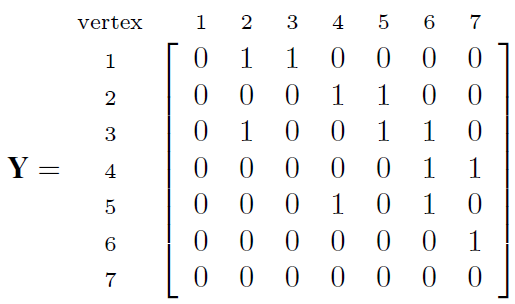(2)

2) Incidence matrix: incidence matrix D is composed in the same way. It express relation R as the D estimation as is shown in Equation (3). We end up with two matrices. The matrix of lists adjacency and occur.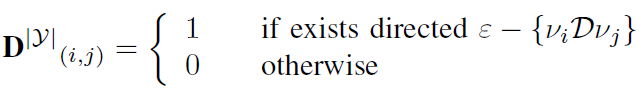(3)

3) Flow sub-weights: let computed flow 𝔽, for our theoretical purpose predefined. To compute proper set of 𝔽̂ε consider Equation (4):(4)

Matrix in Equation (4) is the opposite of an adjacency matrix, because it monitors the quantity of incoming traffic to a node (vector) not an outgoing traffic and is predicted that a Botnet network will generate some overhead traffic from each node. Each node in a P2P Botnet network is both a server and endpoint. It is possible to compute flows as an increasing flow in each transfer point. Practically, it is expected that we will know only 𝔽 which comes from “some source” to the each endpoint.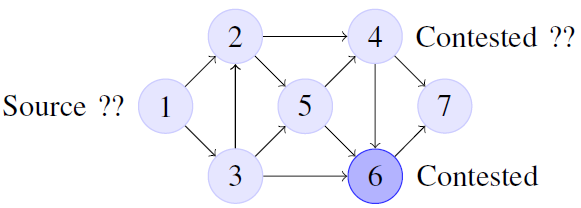Fig. 3: Graph N.

We can use in this case the above calculation NT to solve each 𝔽̂ ⇒ matrix N. It means, that the functional values of the function f are known only at discrete set of points works with scalar product of elements. Therefore it is established weighting function of variables w, where wi = 1; i = 0, 1, ..., n, because we do not have further information on accuracy. Authors, Stastny and Skorpil proposed in article  to solve the scale vector by the Least Mean Square method. In our case, the matrix could have linear dependence, but for the MOO purpose it is listed as the first expression in Equation 5. Assume 𝔽Nε and the residues r = 𝔽Nε, and for the first use we expect that we know all values observed on 𝔽 and also regard to the subject, partial 𝔽p and Np then we can take over basic equation of residua:(5)

4) Probability: as a weight let the probability of a occurrence 𝒟. For this calculation, we selected the Bayesian network approach and Markov’s analysis. Assume only two states:

• state = 1 ; a node is contested by 𝒟1
• state = 0 ; a node is not contested by 𝒟0
Then the serial probability of contest in the network B is:(6)

Parallel is defined as only: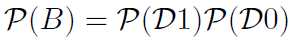(7)

Let us consider at the same time t as a constraint and that each node in N represents sub-input flow from G and is assigned in list Y, may be considered an idea, that the connection between each individual node νij is a two-subsystem and they have in start time t transition function h(t) = λto be contested. For example, take from the figure 3 node 2, which will be detected as contested by D in start time occurrence t, then is λ2,5< λ2,6. If the intensity of “contagion” is constant in time (t1; t2) between any two points, is solved differential equation in condition and the distribution is exponential F(t) = 1−e−λt and let set for the graph N global virtual time T, it can represent proper amount of cycles, then for selected node ν(i,j) with h(𝒟) ↣ ν(i,j): λ(t)h(𝒟) = t∈ T|T−1.

Summary, BN network is created, as is shown in Fig. 4, by lists of node ν, observability O = h(𝒟) of edge " and residual r(Contested; NotContested). If the node is not connected to edge ε, is not connected εi to the residual rj. The probability is set by p(εi | r1, ..., rn).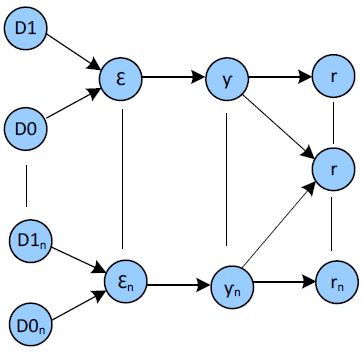Fig. 4: Bayesian Network Model.

Is computed and then created matrix of probabilities p(t) on a space and for the MOO is selected: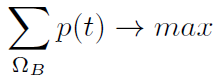(8)

5) Constraints and Objectives: The right computing of constraints is assumed to clear up after correction of mathematical formulas in the sections above. The first constraints to be defined are:

• constraint Y
• constraint in probability
• constraint in 𝔽
• constraint in N;𝒟
Objectives we propose sets up regards to equation (5) and (6) to solve defined steps (6,7).

### CONCLUSION

The focus of this paper is on “Botnet Network”. The main objective is to propose steps to find appearances of a botnet network and illustrate how the NT can be potentially useful, nay, to measure the behavior of a network, but also for other activities such as botnet research and implement multi-objective algorithm to generate an efficient solution. These steps were defined in section A and are part of the mathematical formulas in section C. In a nutshell, two main weights for the calculation are defined. The first weight is the amount of excess flows with the NT combination and a second weight is selected the probability of “spreading” with exponential distribution. Than their costs (minimum) is proposed to search by MOO regards to two main objectives from equation of steps 3 and 4 and the solution is then intentioned to explicitly putt it in the NT. We still have to properly define the limits for multi-objective algorithm. Practical testing will follow. Finally, it would be interesting for further research to test a series of different systems and network form in order to see the efficiency and results between them and improve methods to predict the scope of contested nodes in a network. After this paper’s publishing, we hope to receive more ideas, and corrections from others to improve our conclusion on this subject.

Research described in this paper was financed by the National Sustainability Program under grant LO1401. For the research, infrastructure of the SIX Center was used.

### REFERENCES

 VARDI, Y. Network Tomography: Estimating Source-Destination Traffic Intensities from Link Data. Journal of the American Statistical Association. 1996, vol. 91, issue 433. DOI: 10.2307/2291416.
 UNIVERSITY OF CALIFORNIA’S SAN DIEGO SUPERCOMPUTER CENTER. CAIDA: Center for Applied Internet Data Analysis [online]. 2015 [cit. 2015-01-18]. Available: http://www.caida.org/home/
 RIPE NCC. RIPE Network Coordination Centre: RIPE Labs [online]. 2015 [cit. 2015-01-26]. Available: https://labs.ripe.net/
 POLI, Riccardo, W LANGDON a Nicholas F MCPHEE. A field guide to genetic programming [online]. [S.l.: Lulu Press], 2008, xiv, 233 s. [cit. 2015-01-25]. ISBN 978-1-4092-0073-4. Available: http:// www.gp-field-guide.org.uk/
 GEN, Mitsuo, Runwei CHENG a Lin LIN. Network models and optimization: multiobjective genetic algorithm approach. London: Springer, c2008, xiv, 692 p. ISBN 18-480-0180-0.
 MINARIK, M., STASTNY, J. Recognition of Randomly Deformed Objects. In MENDEL 2008, 14th International Conference on Soft Computing. Brno University of Technology, 2008, pp. 275-280, ISBN 978-80- 214-3675-6
 MEMON, Rashida A., Sameer QAZI a Adnan A. FAROOQUI. Network tomography using genetic algorithms. TENCON 2012 IEEE Region 10 Conference [online]. IEEE, 2012, s. 1-6 [cit. 2015-01-14]. DOI: 10.1109/TENCON.2012.6412313. Available: http://ieeexplore.ieee. org/lpdocs/epic03/wrapper.htm?arnumber=6412313
 STASTNY, J., SKORPIL, V. Analysis of Algorithms for Radial Basis Function Neural Network. Personal Wireless Communications, Springer, 2007, volume 245, pp. 54-62, ISSN 1571- 5736, ISBN 978-0-387-74158- 1

Autor:        V. Oujezský, V. Škorpil, M. Jurčík
Pracoviště: Vysoké učení technické v Brně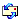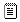Projekty a aktuality
01.03.2012: PROJEKT
Výzkum a vývoj nového komunikačního systému s vícekanálovým přístupem a mezivrstvovou spoluprací pro průmyslové aplikace TA02011015

01.01.2012: PROJEKT
Vývoj adaptabilních datových a procesních systémů pro vysokorychlostní, bezpečnou a spolehlivou komunikaci v extrémních podmínkách VG20122014095

09.10.2010: PROJEKT
Výzkum a vývoj datového modulu 10 Gbit/s pro optické a mikrovlnné bezdrátové spoje, FR-TI2/621

09.01.2010: PROJEKT
Sítě s femtobuňkami rozšířené o řízení interference a koordinaci informací pro bezproblémovou konektivitu, FP7-ICT-2009-4 248891

09.11.2008: PROJEKT
Ochrana člověka a techniky před vysokofrekvenčním zářením, FI-IM5/202

20.06.2008: Schválení
Radou pro výzkum a vývoj jako recenzovaný časopis

01.04.2007: PROJEKT
Pokročilá optimalizace návrhu komunikačních systémů pomocí neuronových sítí, GA102/07/1503

01.07.2006: Doplnění sekce pro registrované

12.04.2005: Zavedeno recenzování článků

30.03.2005: Výzkumný záměr
Výzkum perspektivních informačních a komunikačních technologií MSM6840770014

29.11.2004: Přiděleno ISSN

04.11.2004: Spuštění nové podoby Access serveru

18.10.2004: PROJEKT
Optimalizace přenosu dat rychlostí 10 Gbit/s, GA102/04/0773

04.09.2004: PROJEKT
Specifikace kvalitativních kritérií a optimalizace prostředků pro vysokorychlostní přístupové sítě, NPV 1ET300750402

04.06.2004: PROJEKT
Omezující faktory při širokopásmovém přenosu signálu po metalických párech a vzájemná koexistence s dalšími systémy, GA102/03/0434Tento web site byl vytvořen prostřednictvím phpRS - redakčního systému napsaného v PHP jazyce.
Na této stránce použité názvy programových produktů, firem apod. mohou být ochrannými známkami
nebo registrovanými ochrannými známkami příslušných vlastníků.